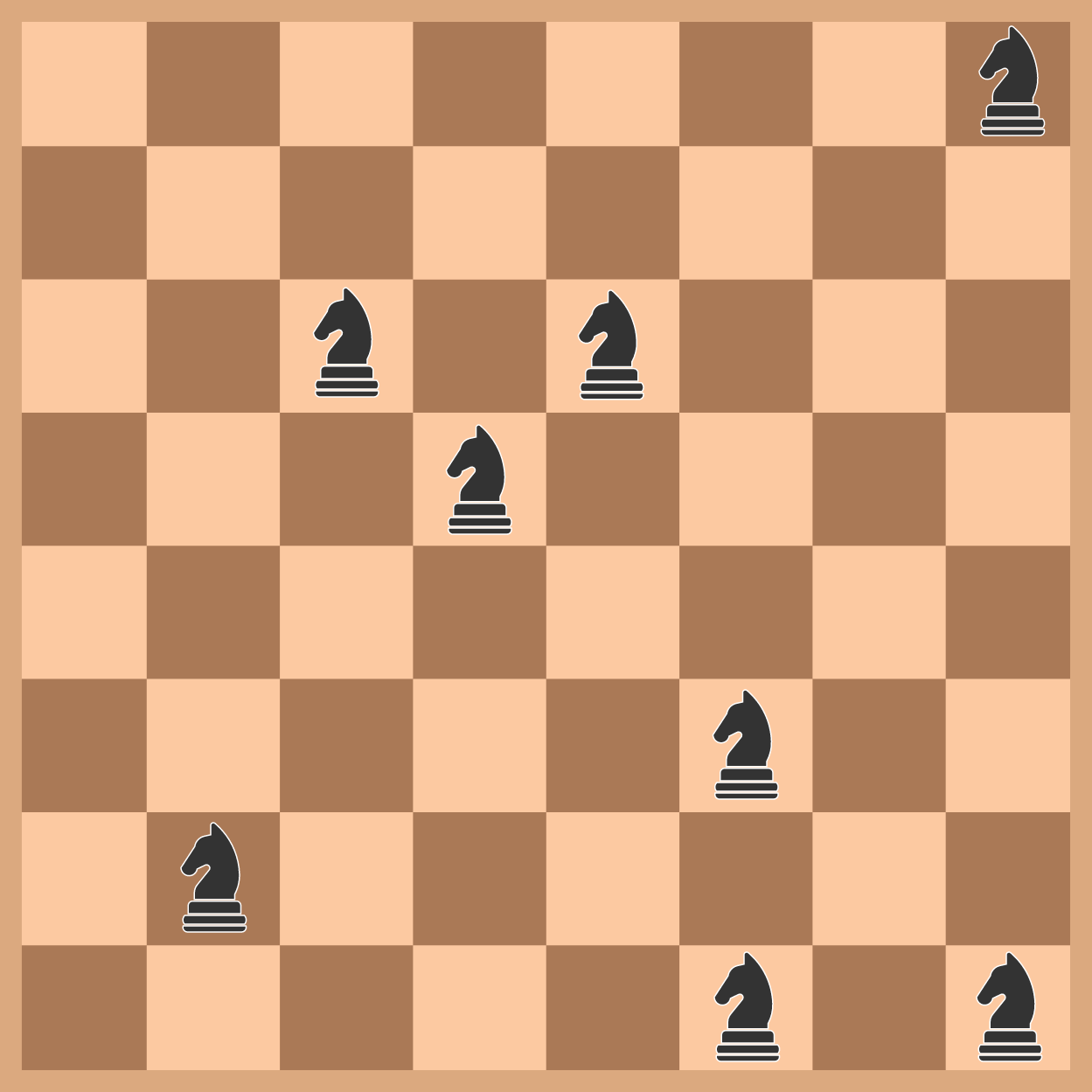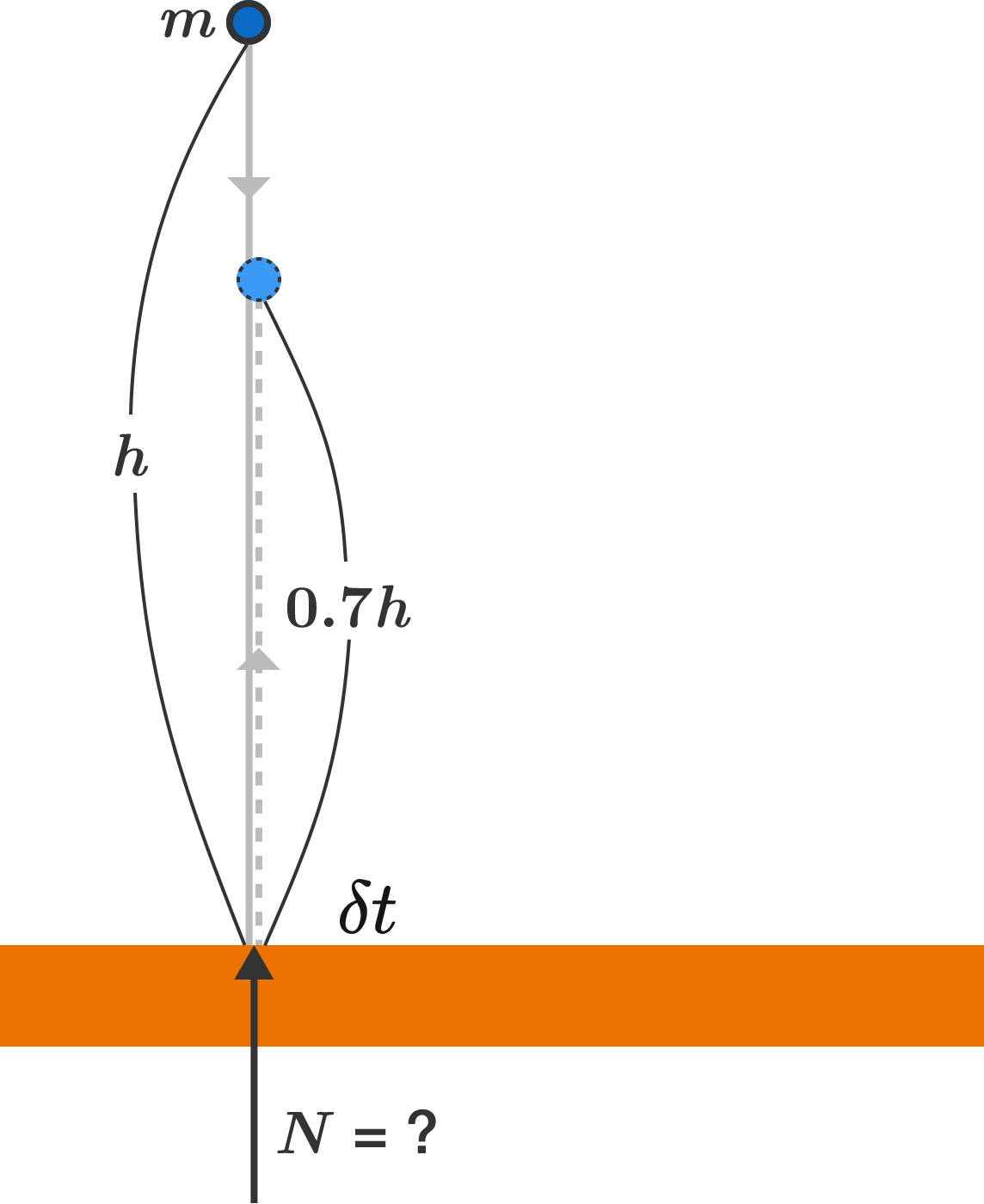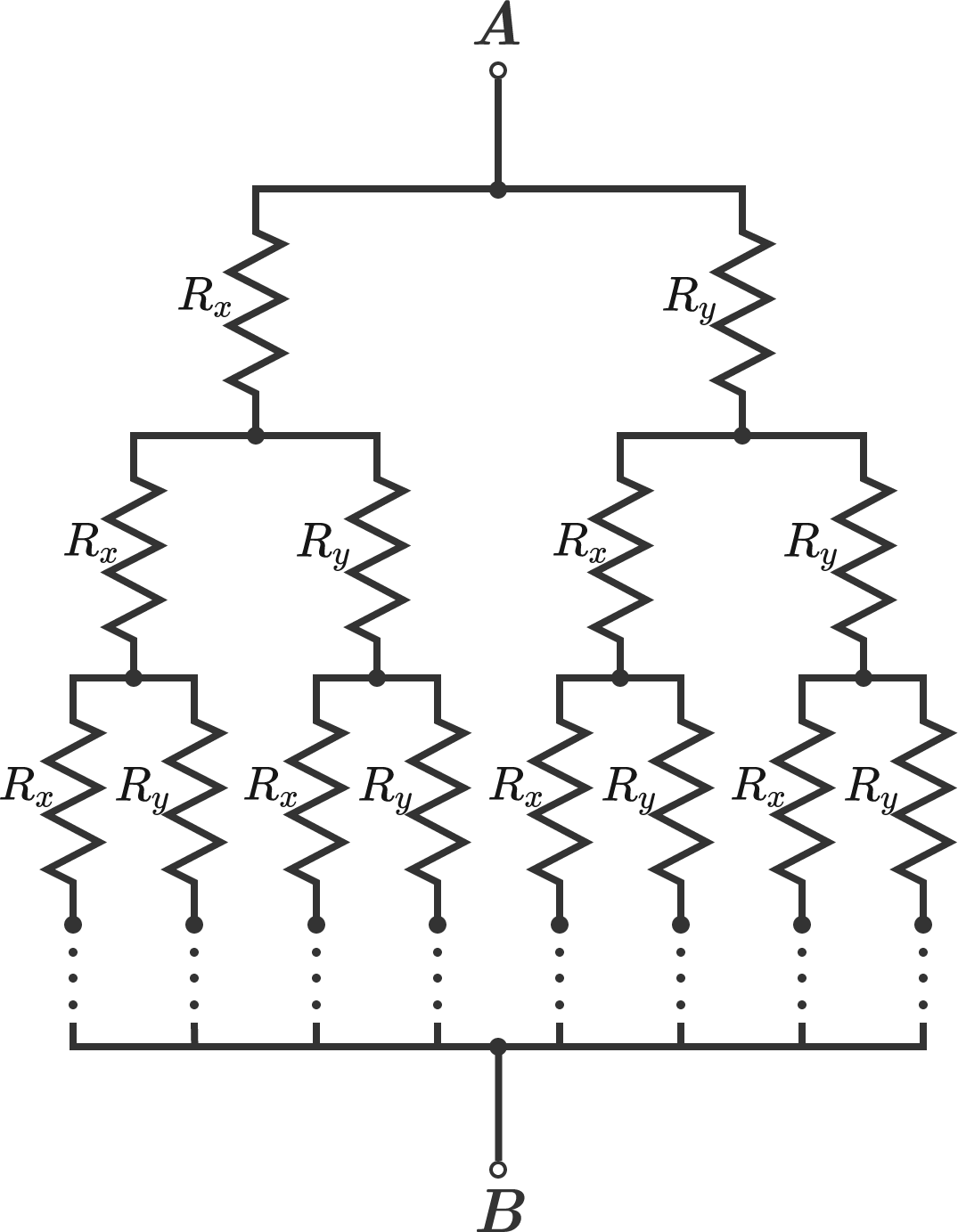# Problems of the Week

Contribute a problem

# 2017-08-07 Intermediate

What is the maximum number of chess knights that you can place on a chessboard such that none of them are attacking each other?Note: The knights in question have no allegiance to white or black. The picture above shows knights which cannot attack each other in one move.

3 frogs are sitting on 3 of four lily pads in a line, leaving one unoccupied, as shown below:A frog can only move to the empty lily pad either

• by jumping to it if the frog is immediately next to the empty lily pad, or
• by leaping over one frog if the frog is two seats away from the empty lily pad.

What is the least number of moves required in total for all the frogs to arrange themselves in the following configuration?A ball of mass $m$ is dropped from some height in a (uniform) gravitational field of $g$. It reaches the ground and bounces directly upward, to 70% of its original height. During the bounce, the ball is in contact with the ground for a small, non-zero time interval of $\delta t$.

What can be said about the normal force between the ball and ground during the interval $\delta t?$This resistive network forms an infinite binary tree--every branch splits into two new branches, where the new branch that goes to the left has a resistance of $R_x$, and the one that goes to the right has a resistance of $R_y$.

Suppose that after going down $n$ levels, all of the branches are connected to a single node, $B$. As $n$ approaches infinity, what will be the equivalent resistance between nodes $A$ and $B?$

Consider the sum of the reciprocals of every positive integer that doesn't contain the digit 2:

$\frac{1}{1} + \frac{1}{3} + \frac 14 + \cdots + \frac{1}{10} + \frac{1}{11} + \frac{1}{13} +\cdots+ \frac{1}{19} + \frac{1}{30} + \frac 1{31} \cdots.$

Does this sum converge?

×